Learn to click the perfect selfie Learn to click the perfect selfie

# ICSE Class 10 Chemistry Question Paper Solution 2017

ICSE Class 10 Chemistry Previous year question papers give an idea on the paper pattern, important topics from which questions are expected to be asked in the exam. So, it becomes crucial for students to solve the ICSE Class 10th previous year papers for board exam preparation. We have provided the ICSE Class 10 Chemistry Question Paper 2017 Solution to help students with their exam preparation. The answers provided in the solution pdf are based on the step marking as per the board exam marking scheme. If a student expresses the answer in the same manner as mentioned in the solution pdf, then he/she will surely score high marks in the exam.

The ICSE Class 10 Chemistry 2017 exam was conducted on 3rd April 2017. The exam started at 11 am and students were allotted 2 hours of time duration to finish the paper. Students can download the ICSE Class 10 Chemistry Question Paper Solution 2017 PDF from the link below.

Students can have a look at the ICSE Class 10 Chemistry Question Paper Solution 2017 below: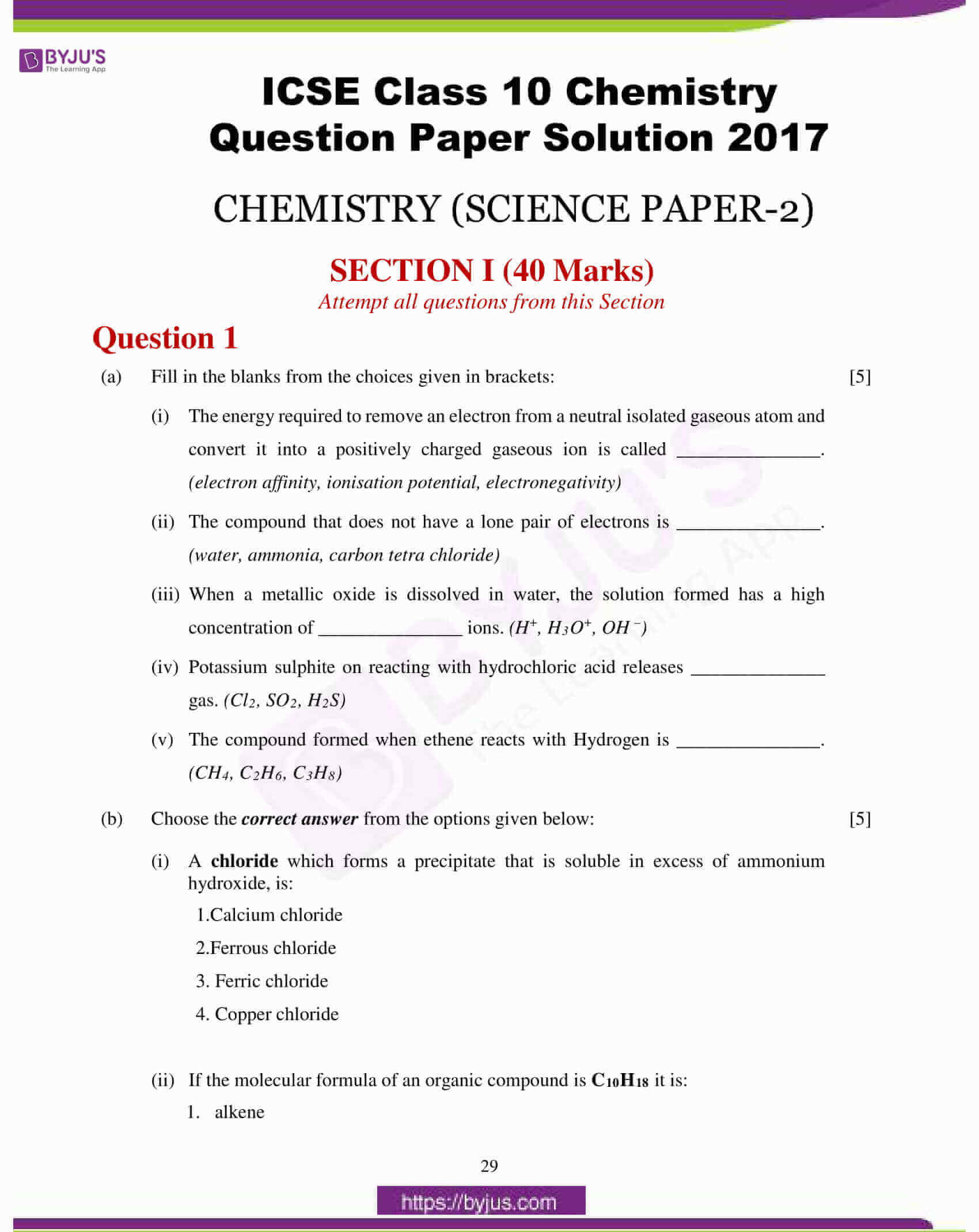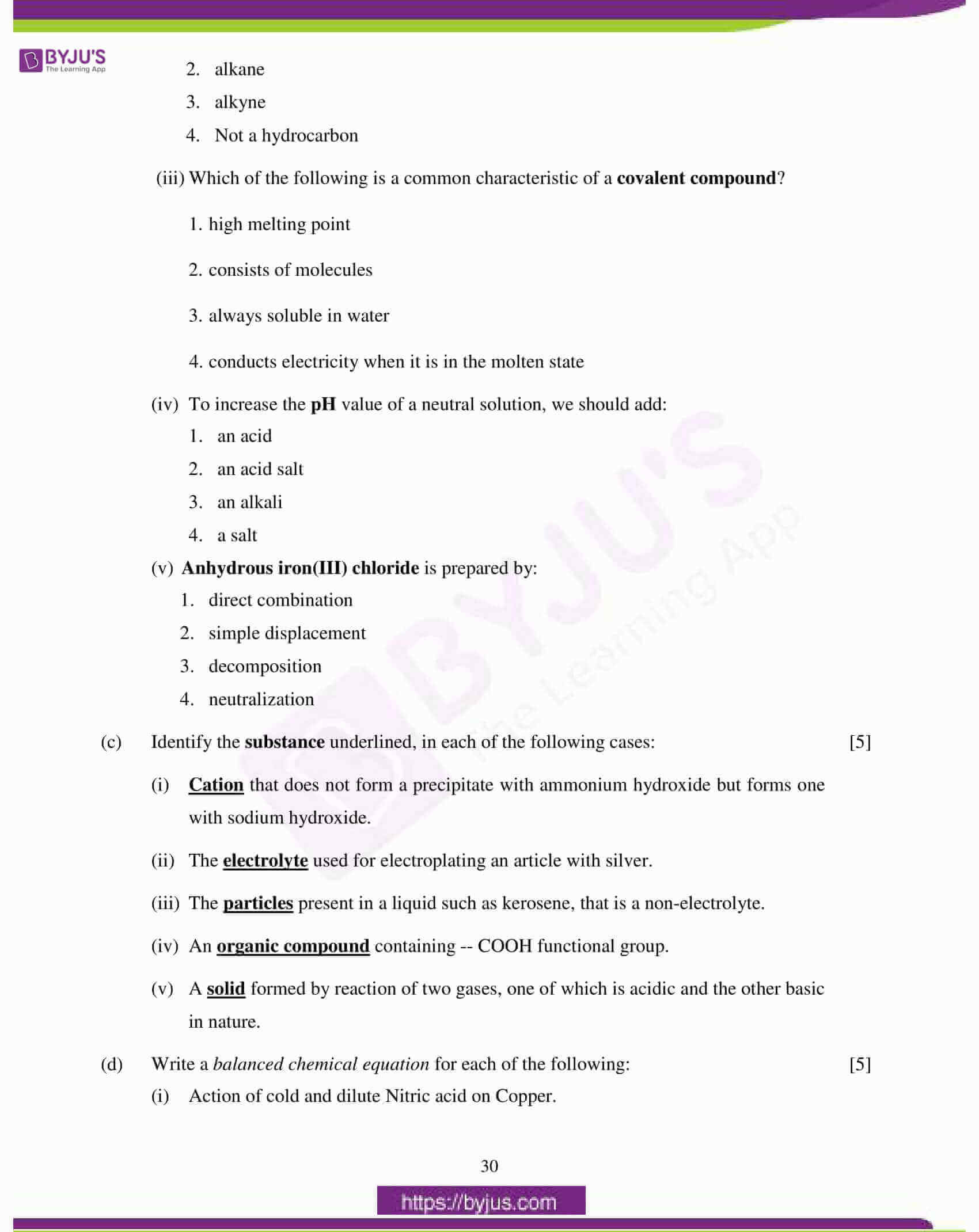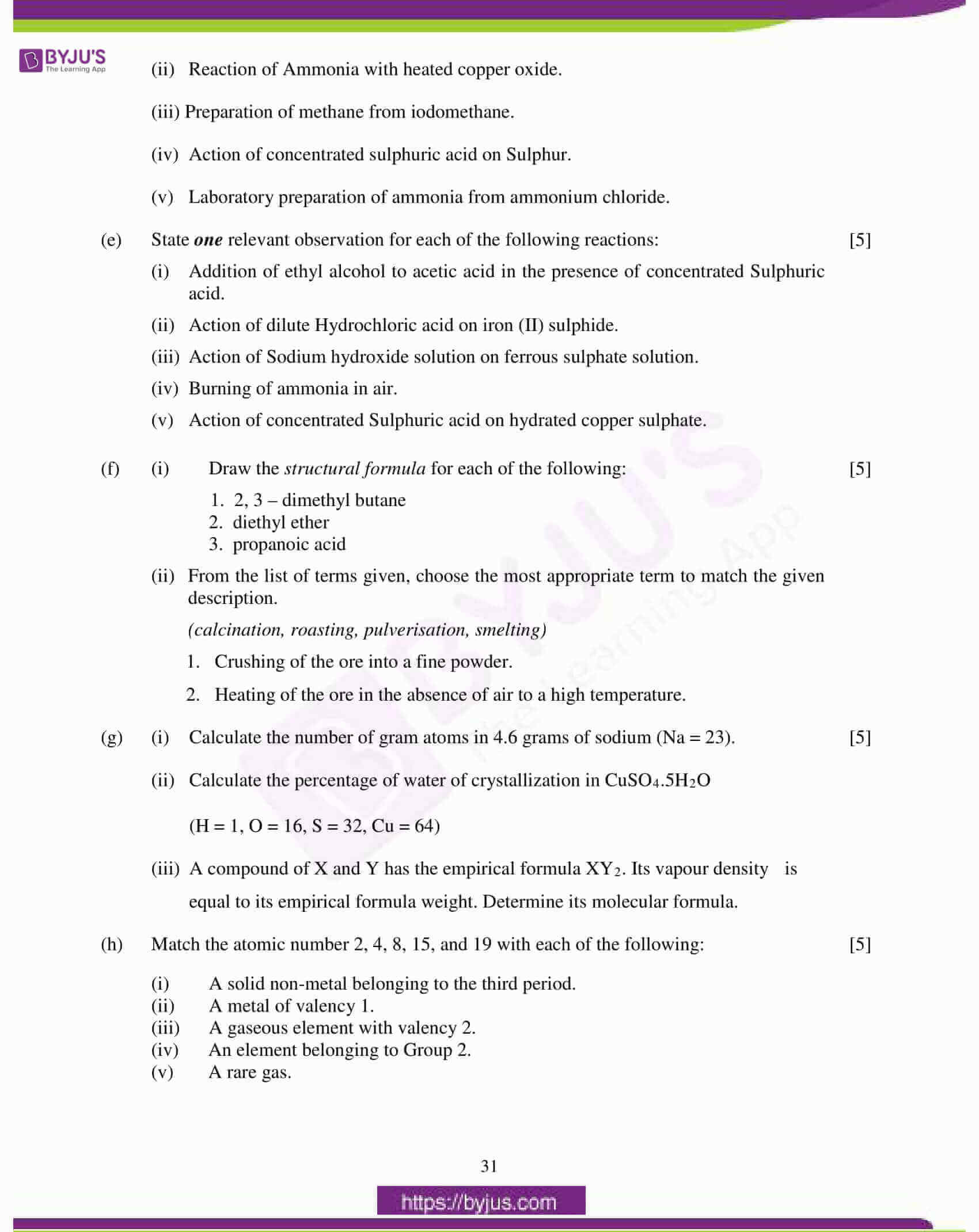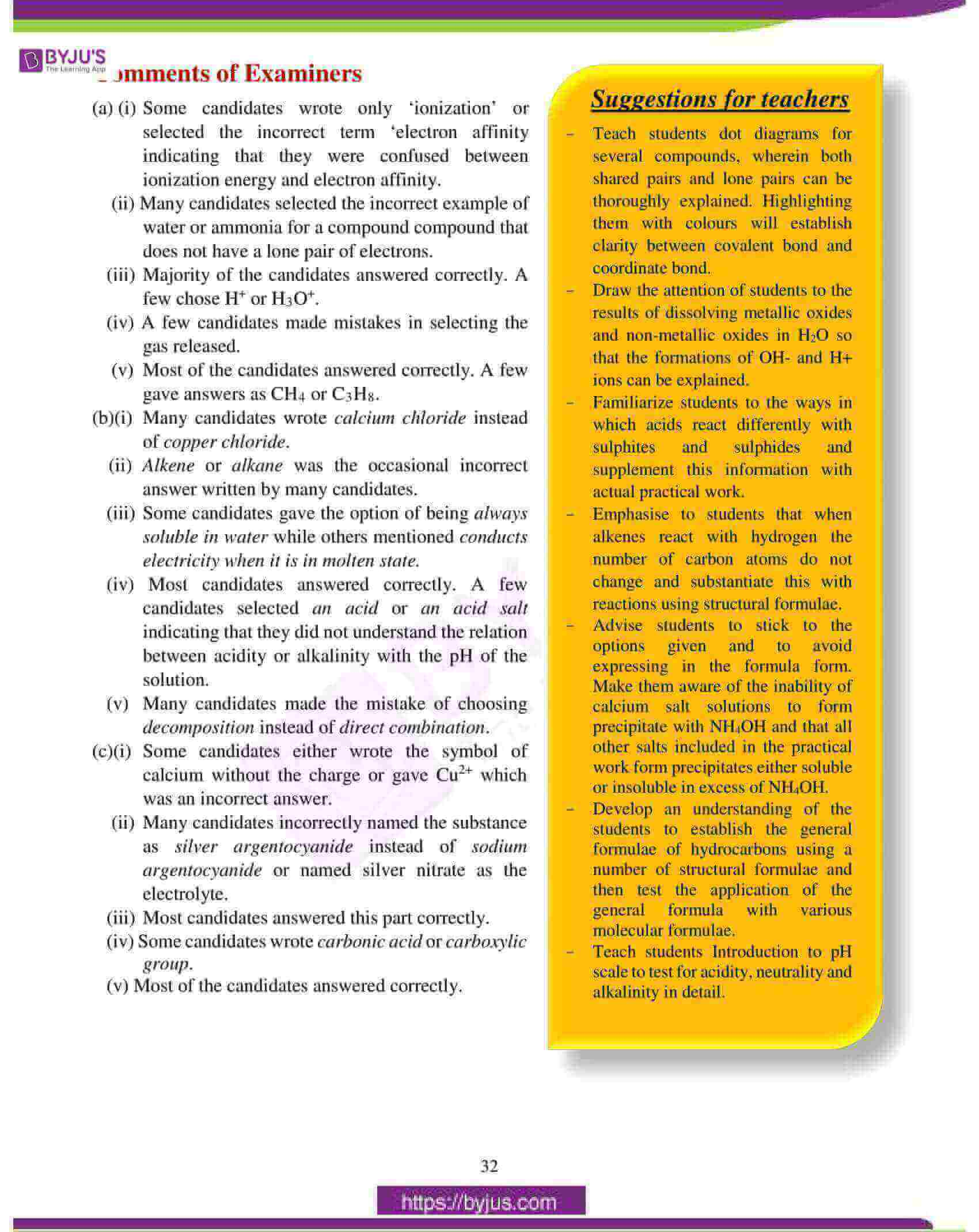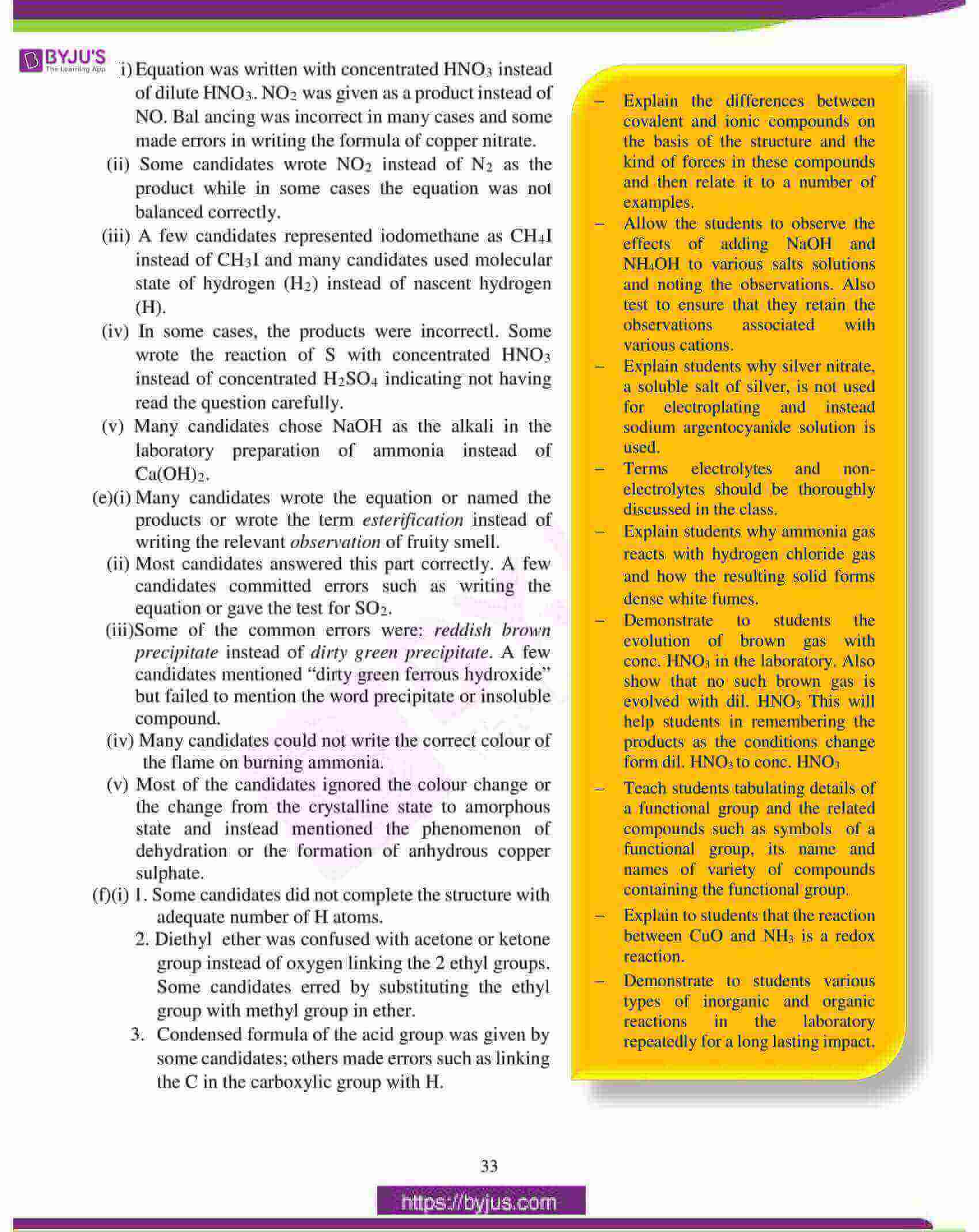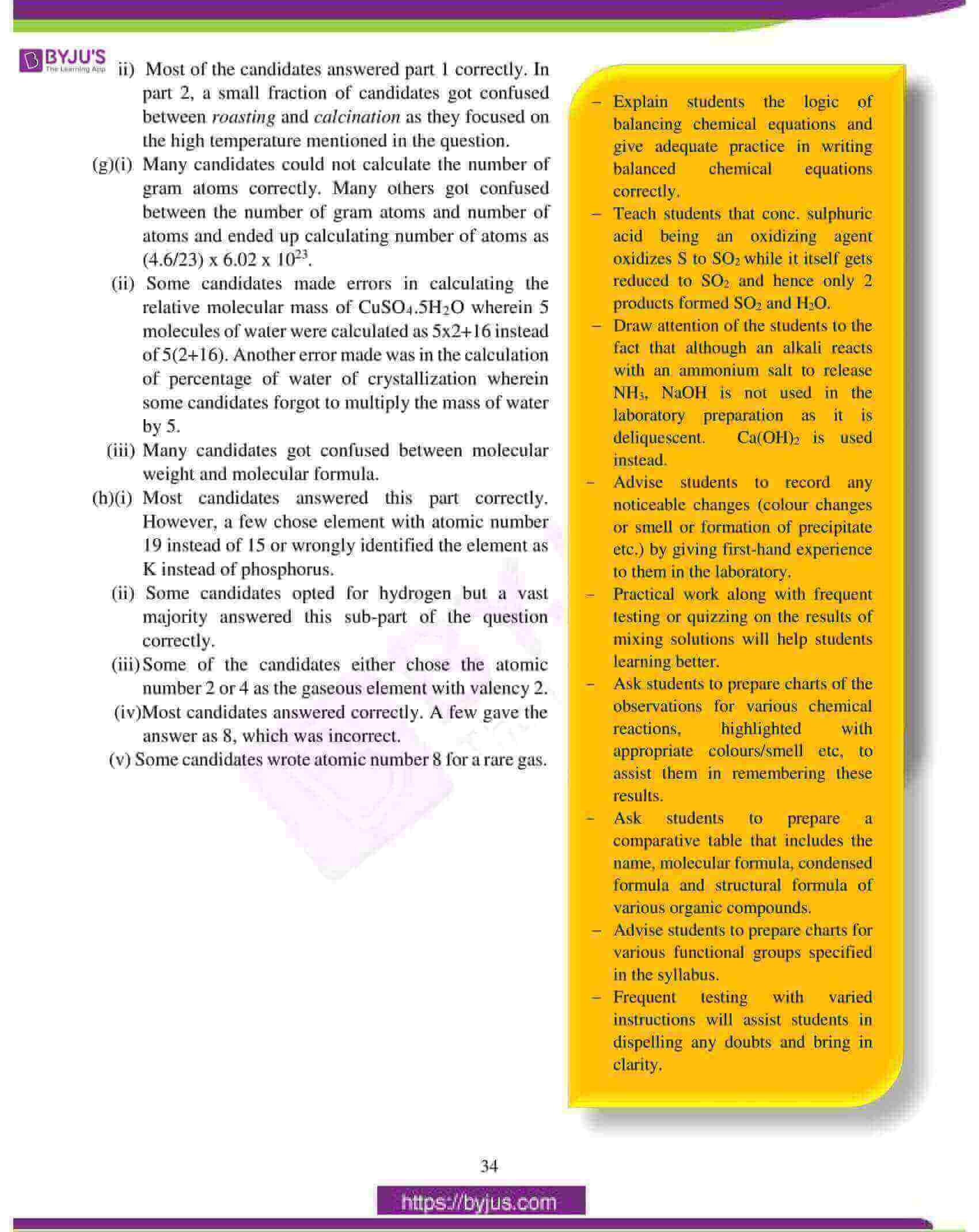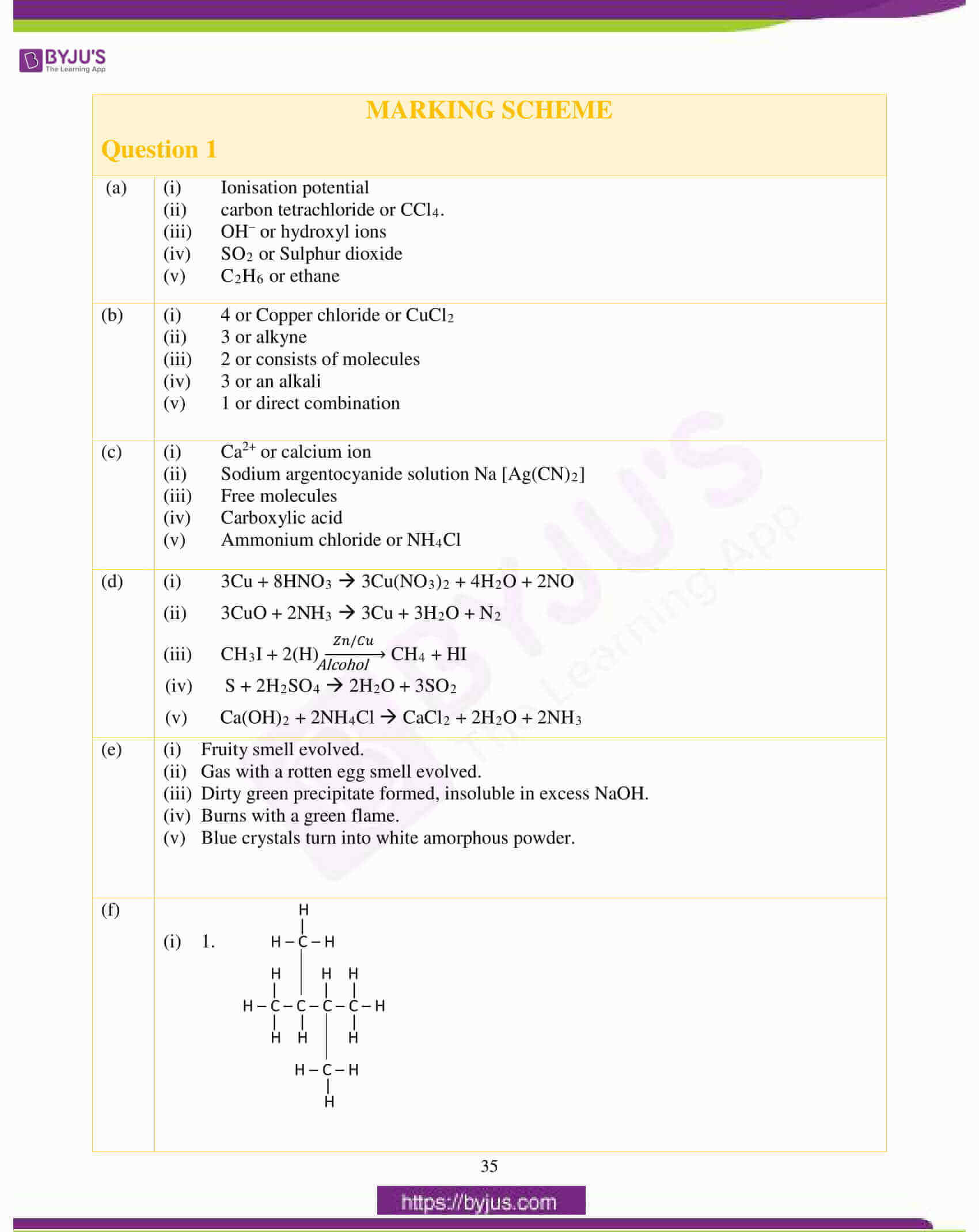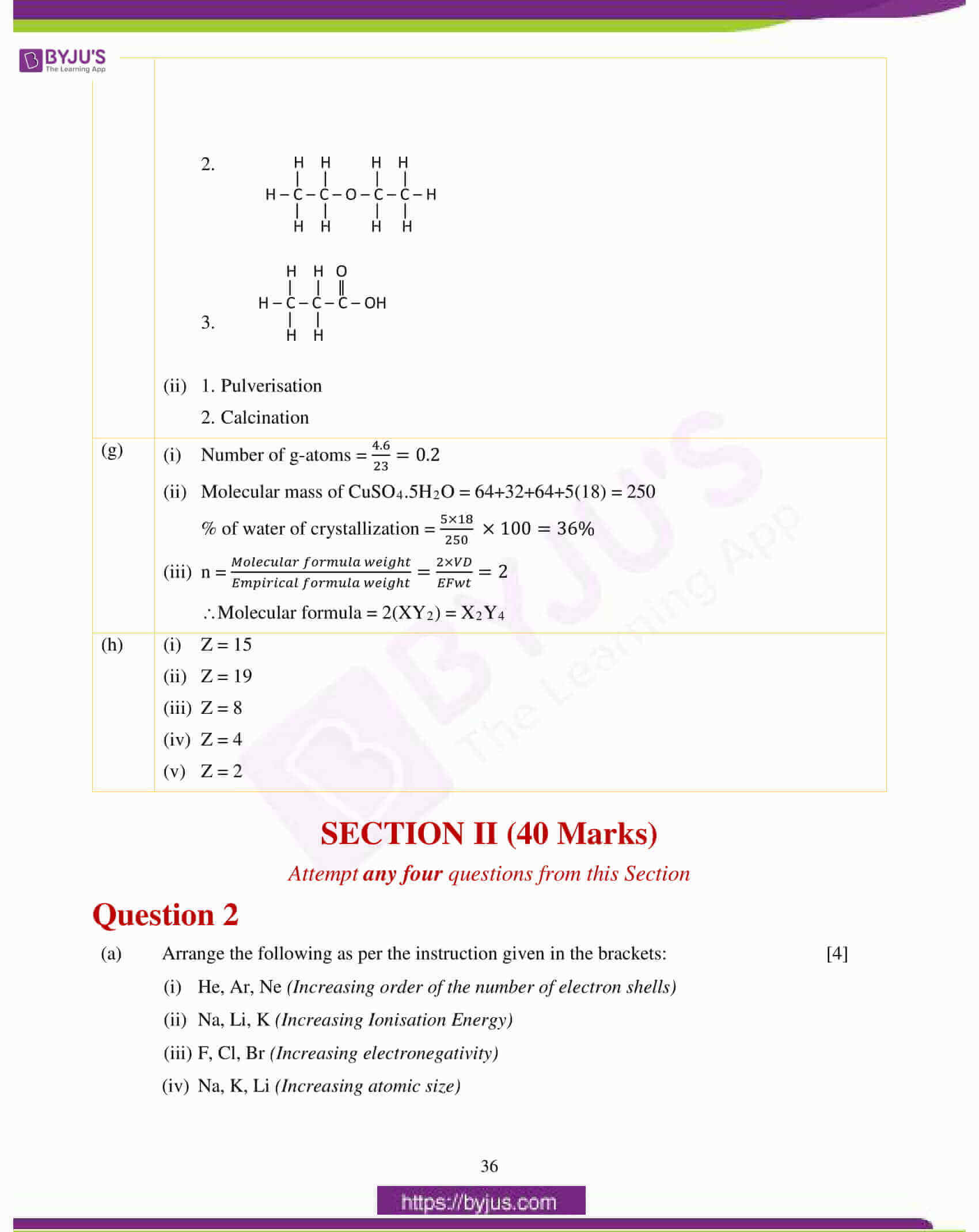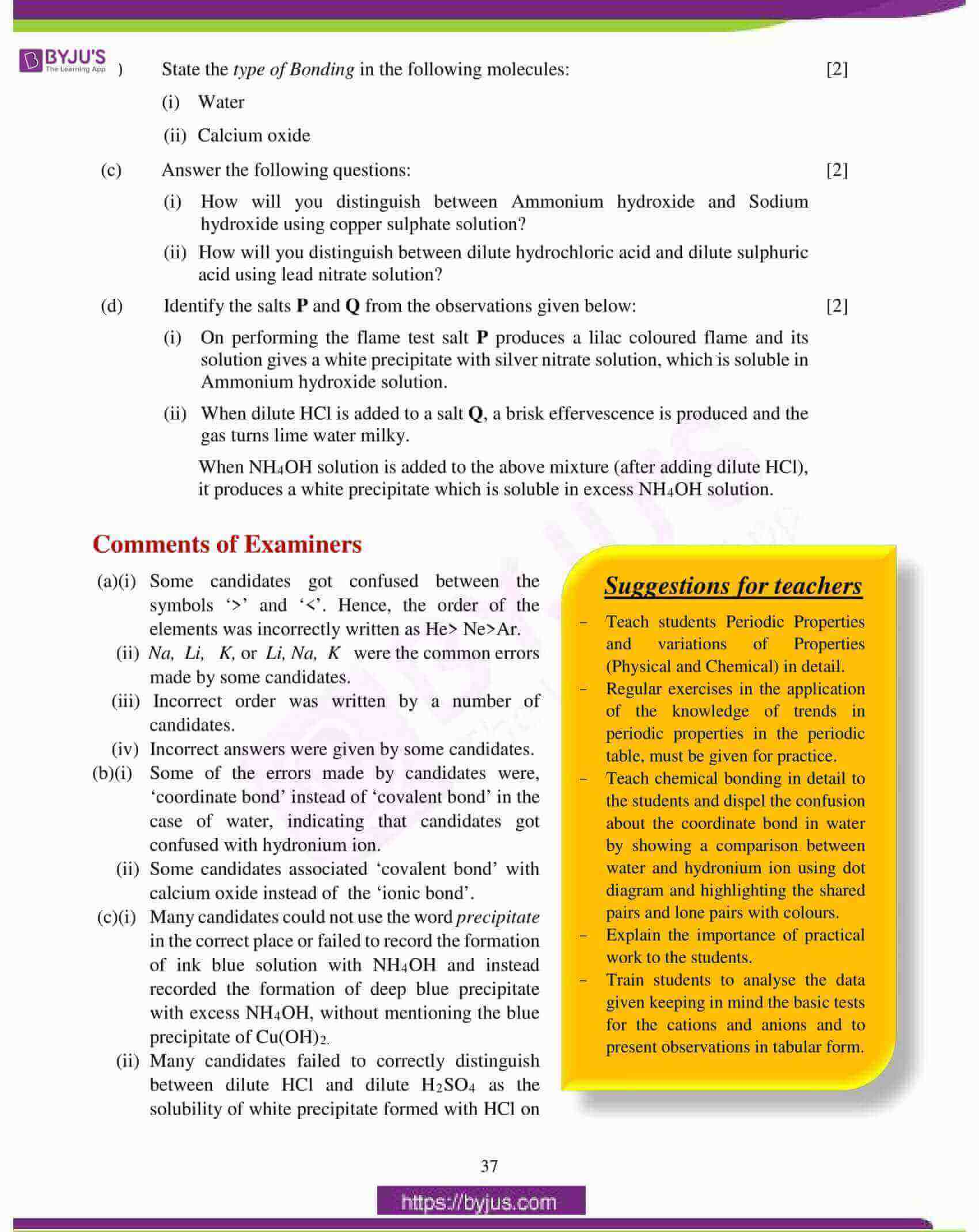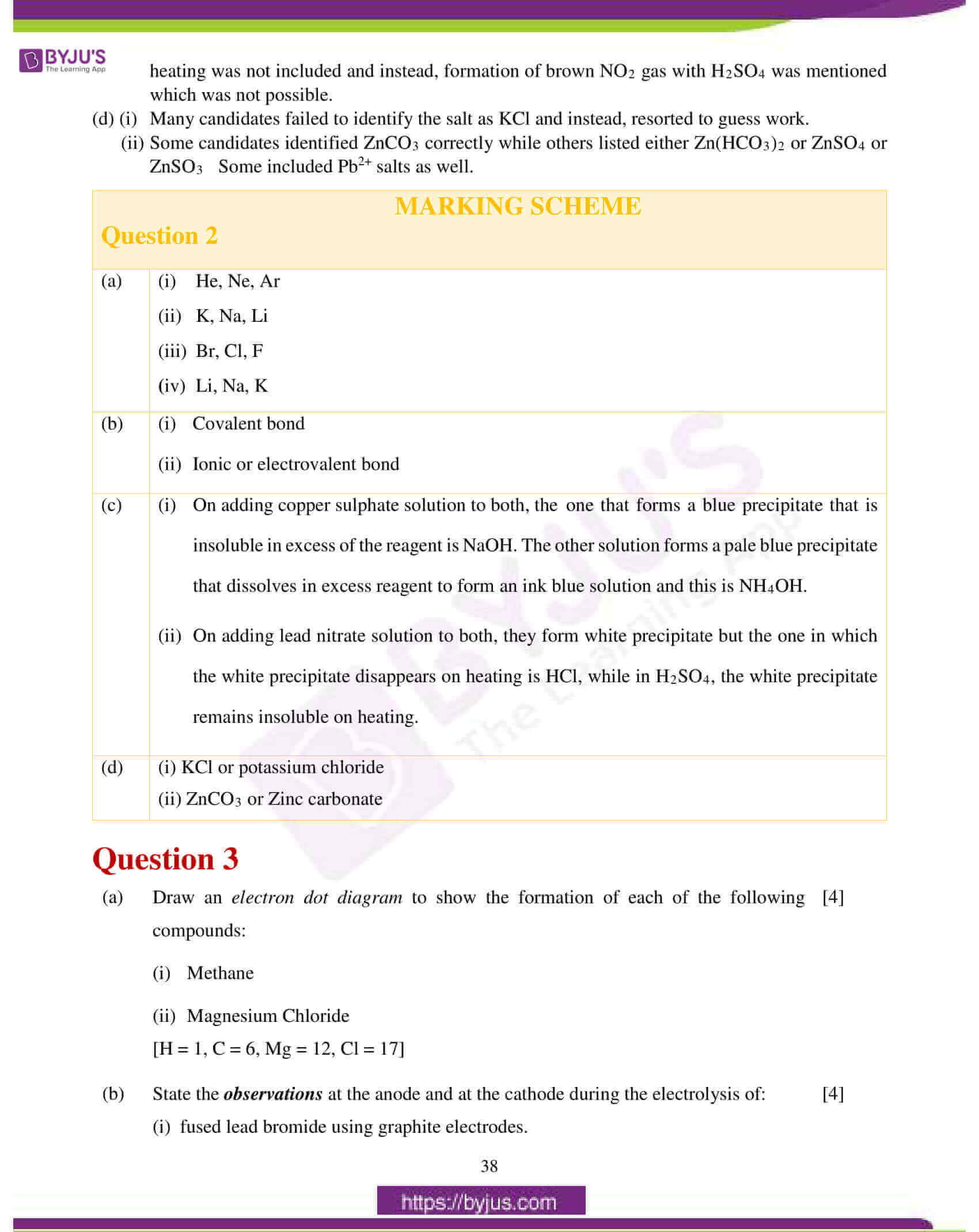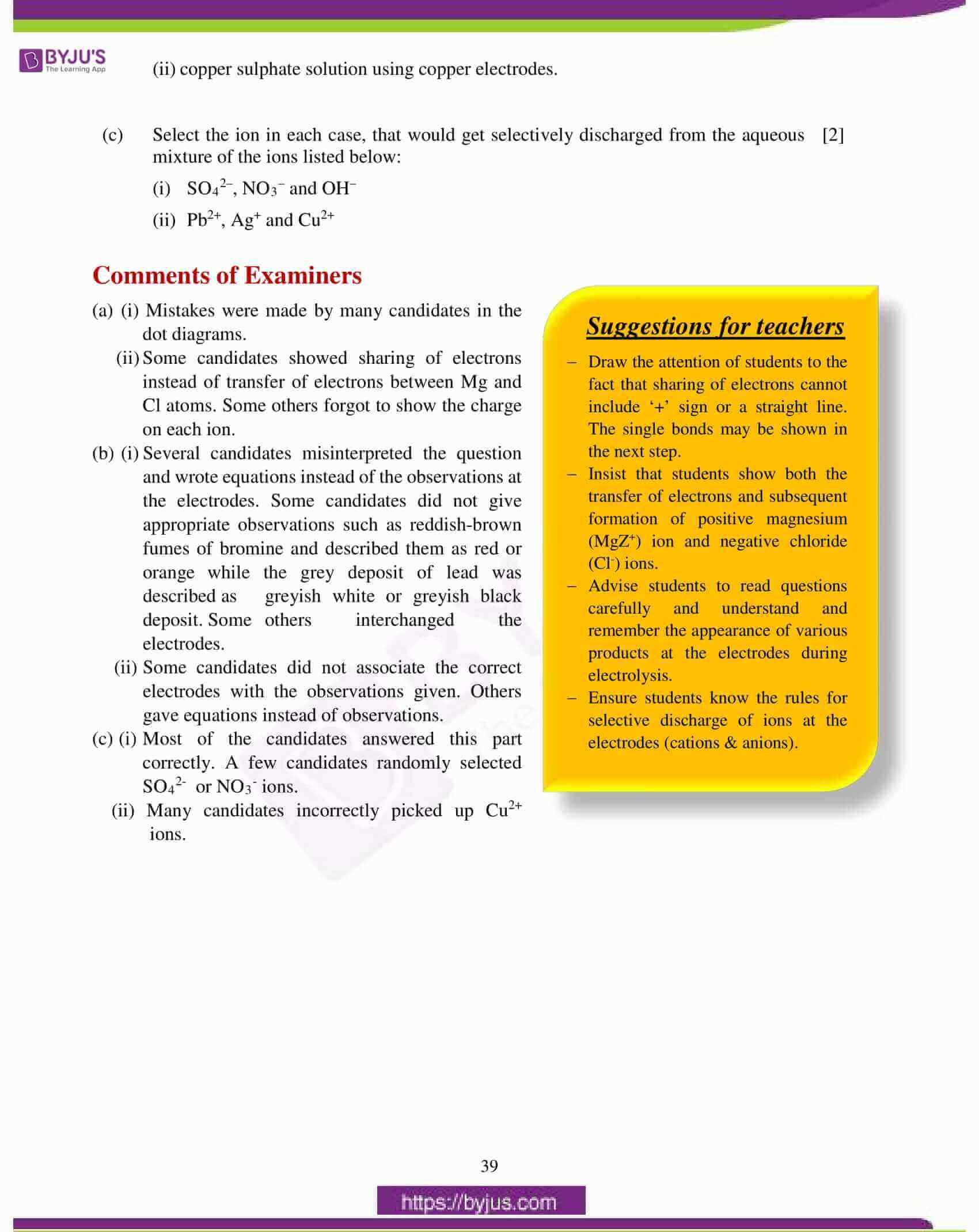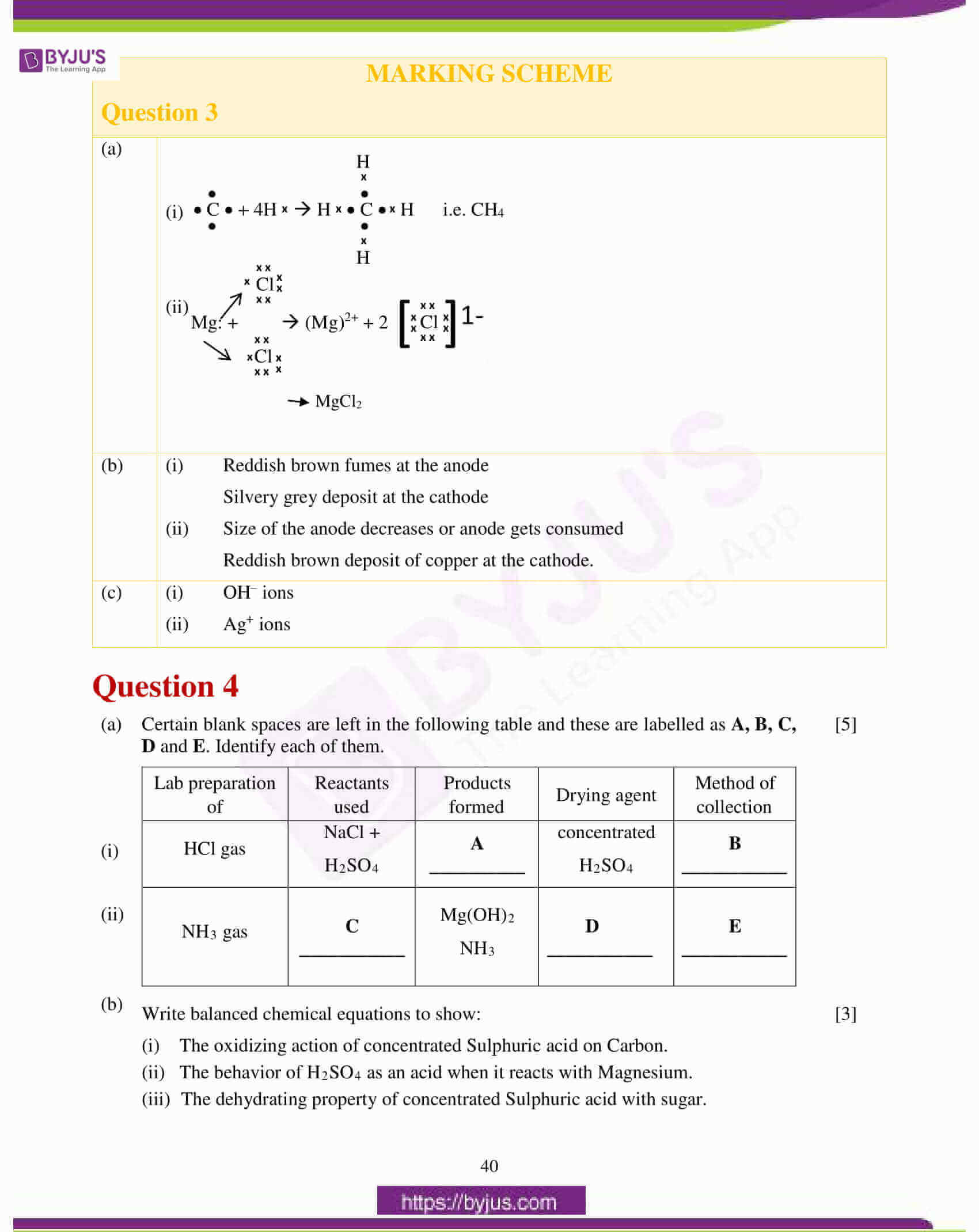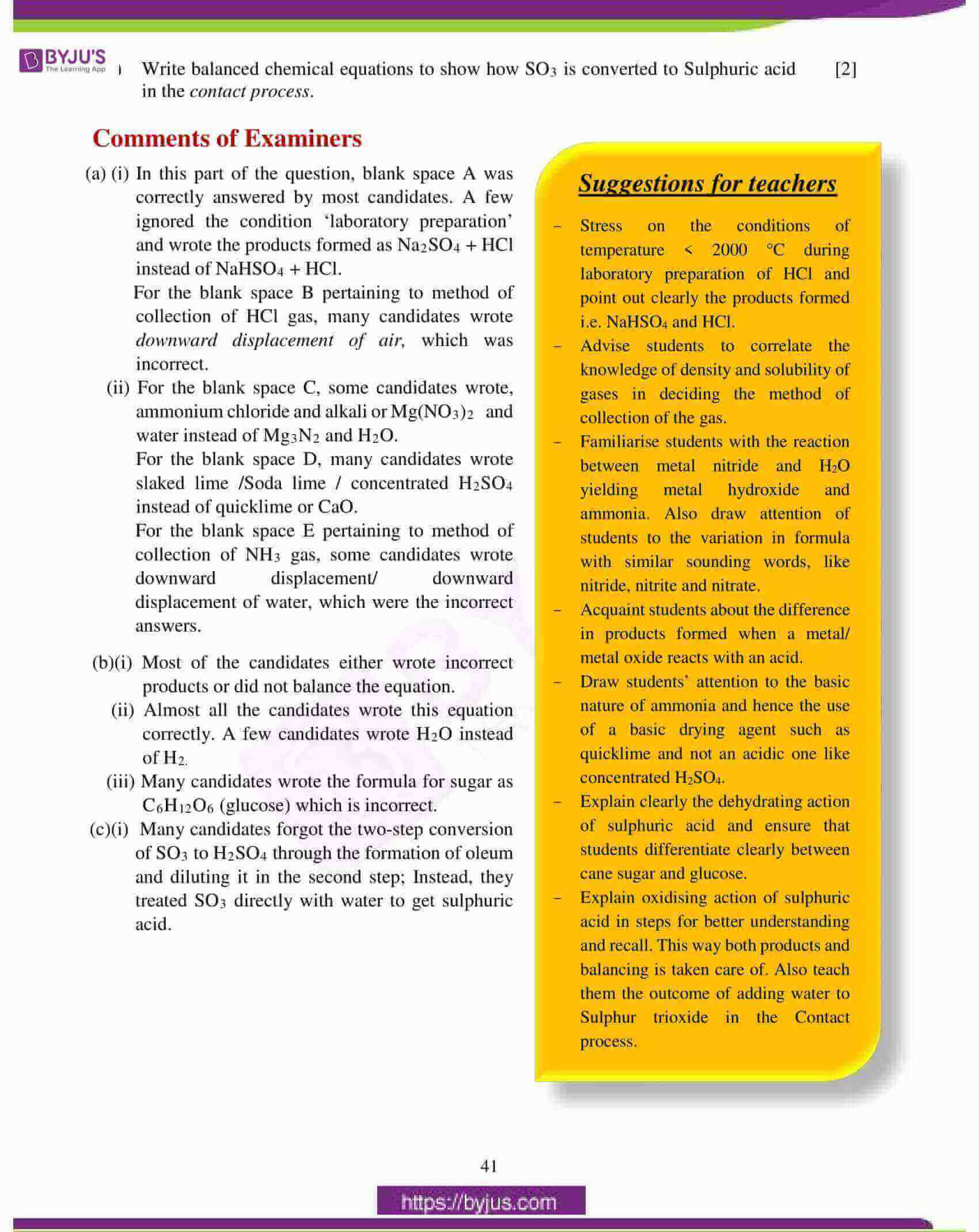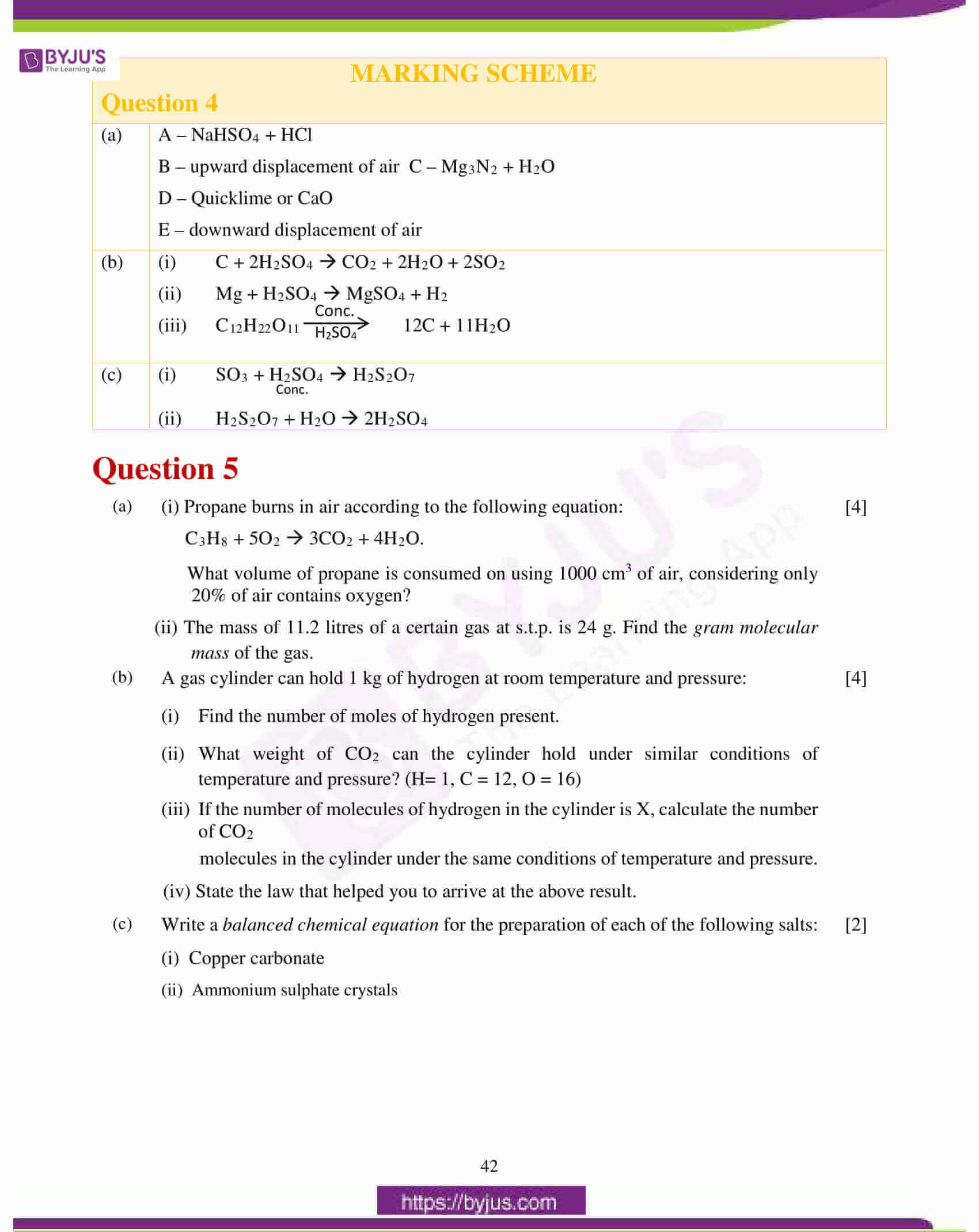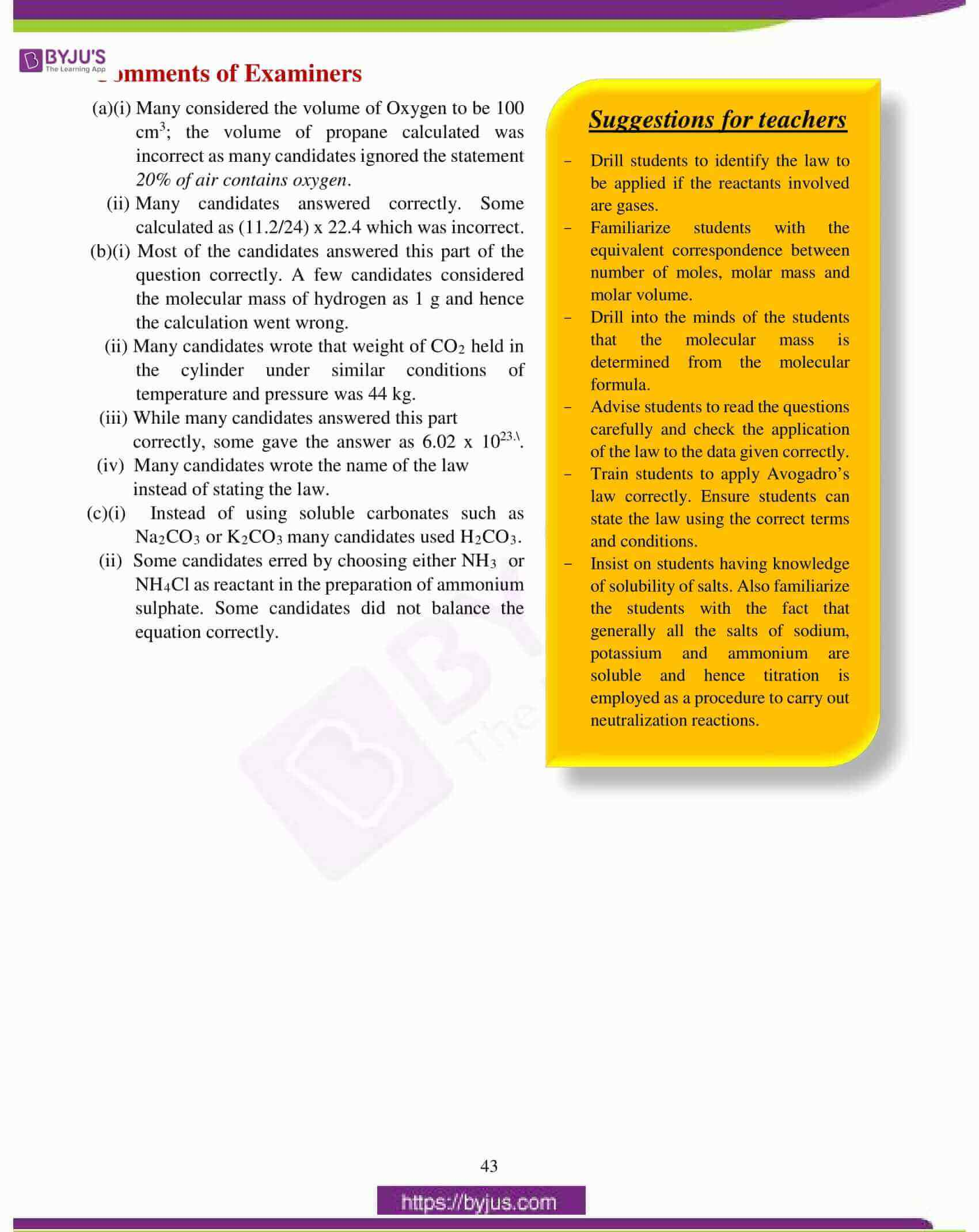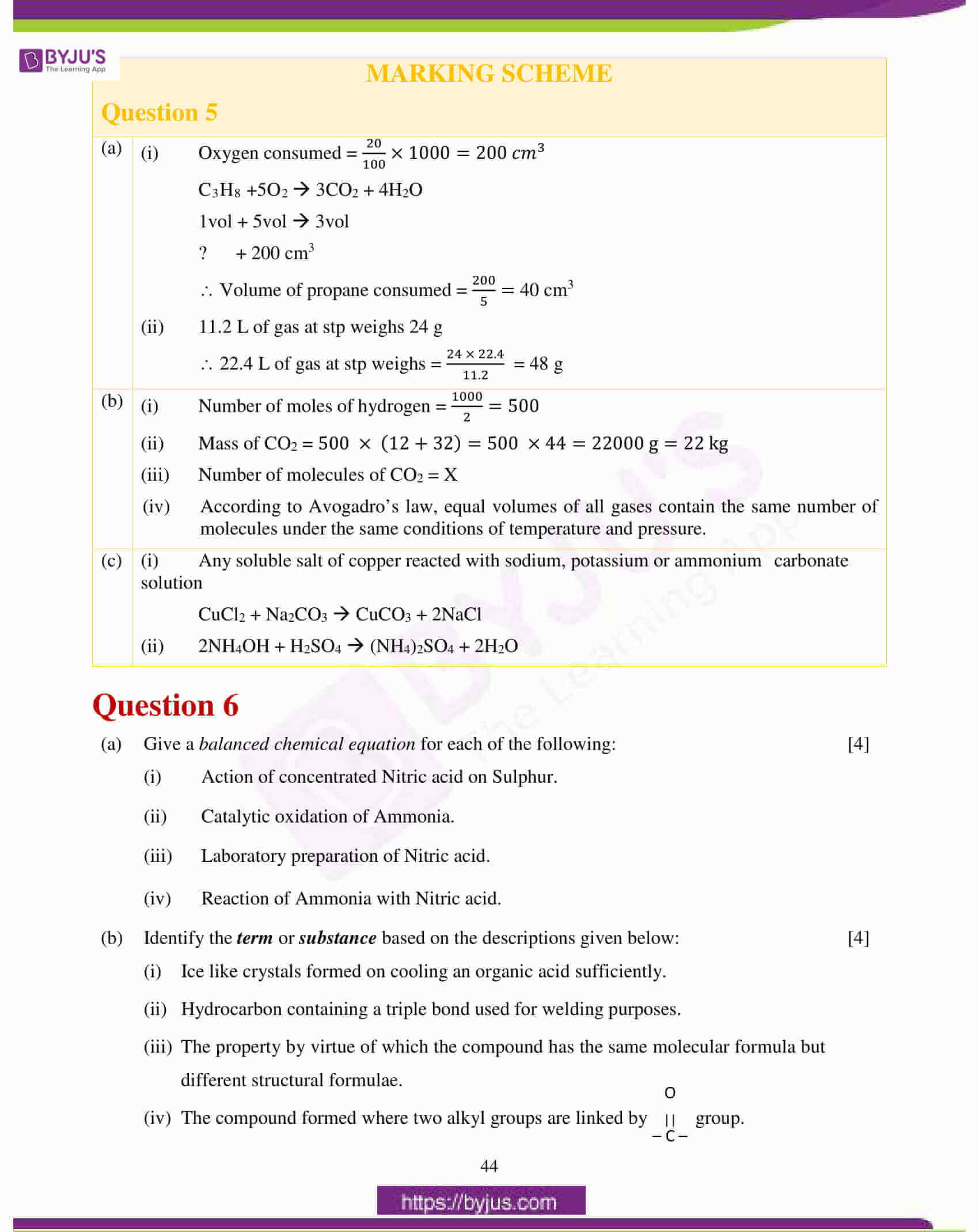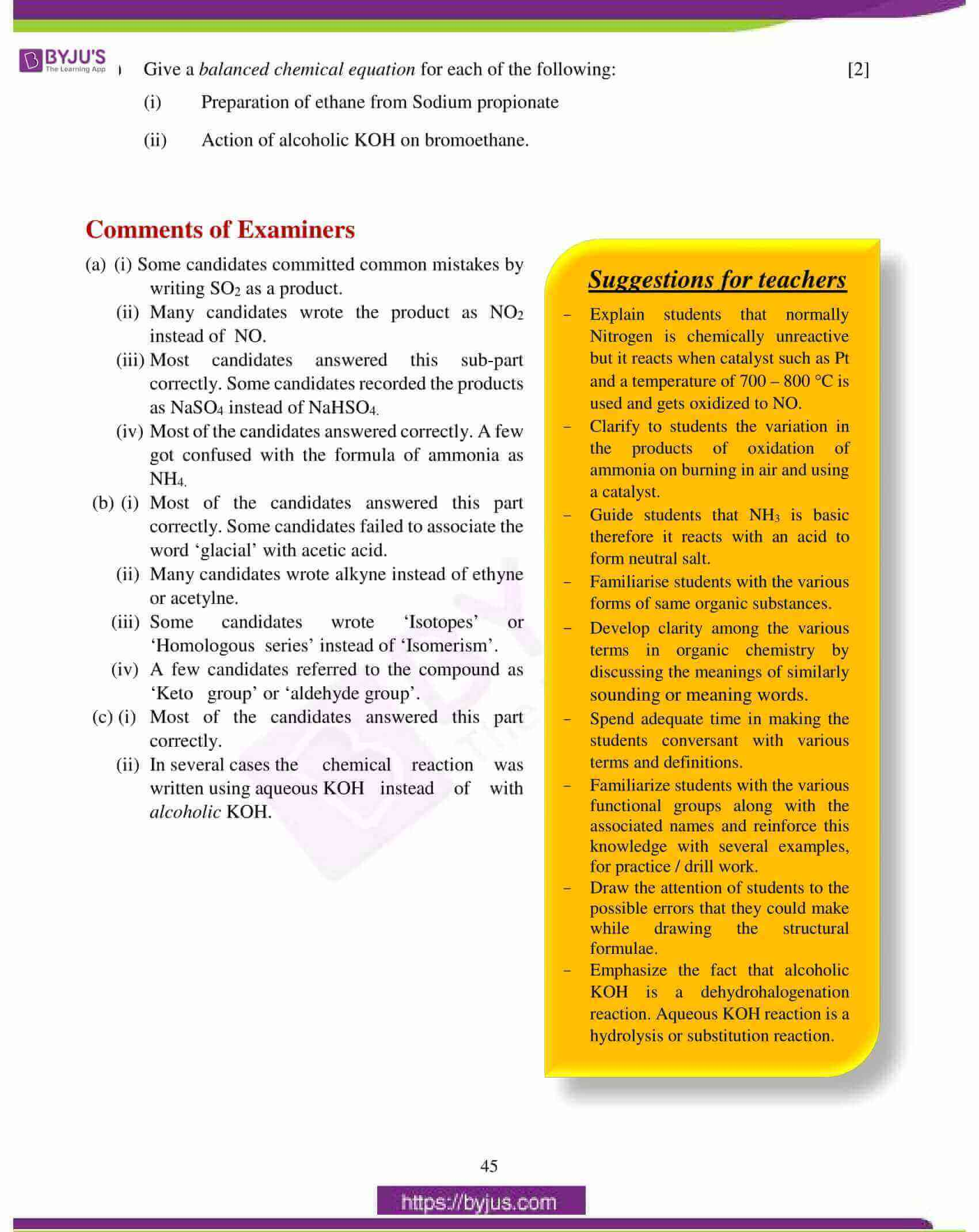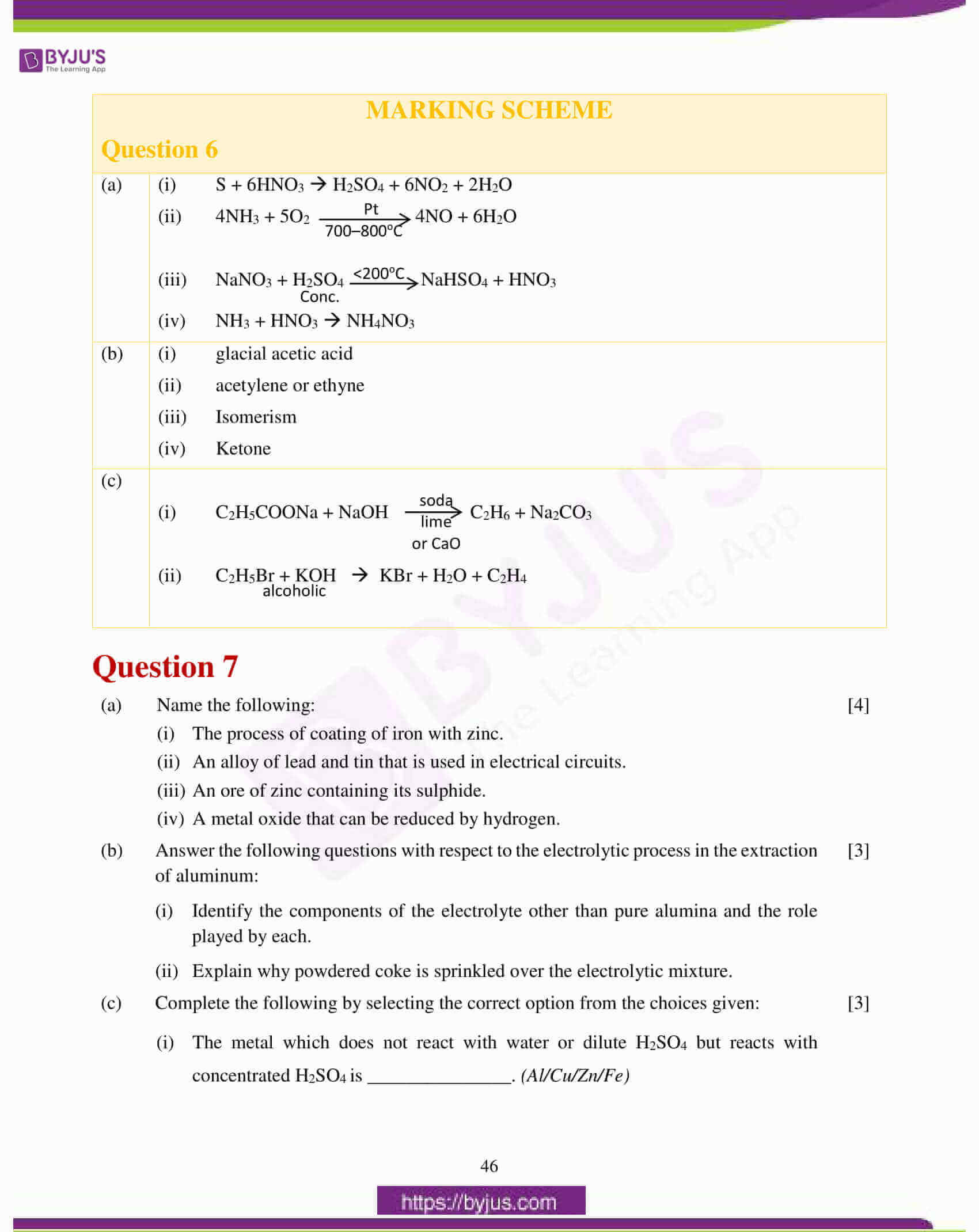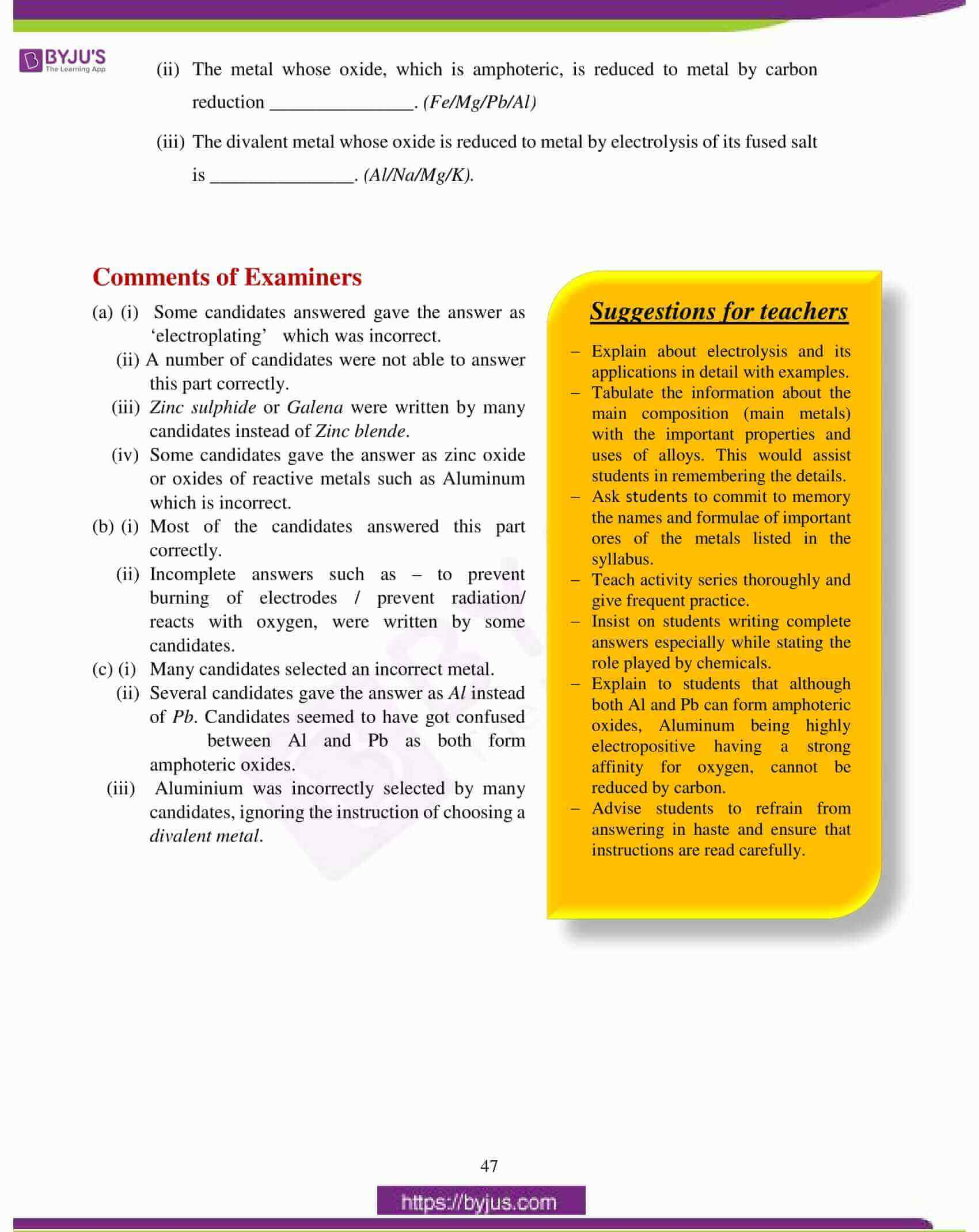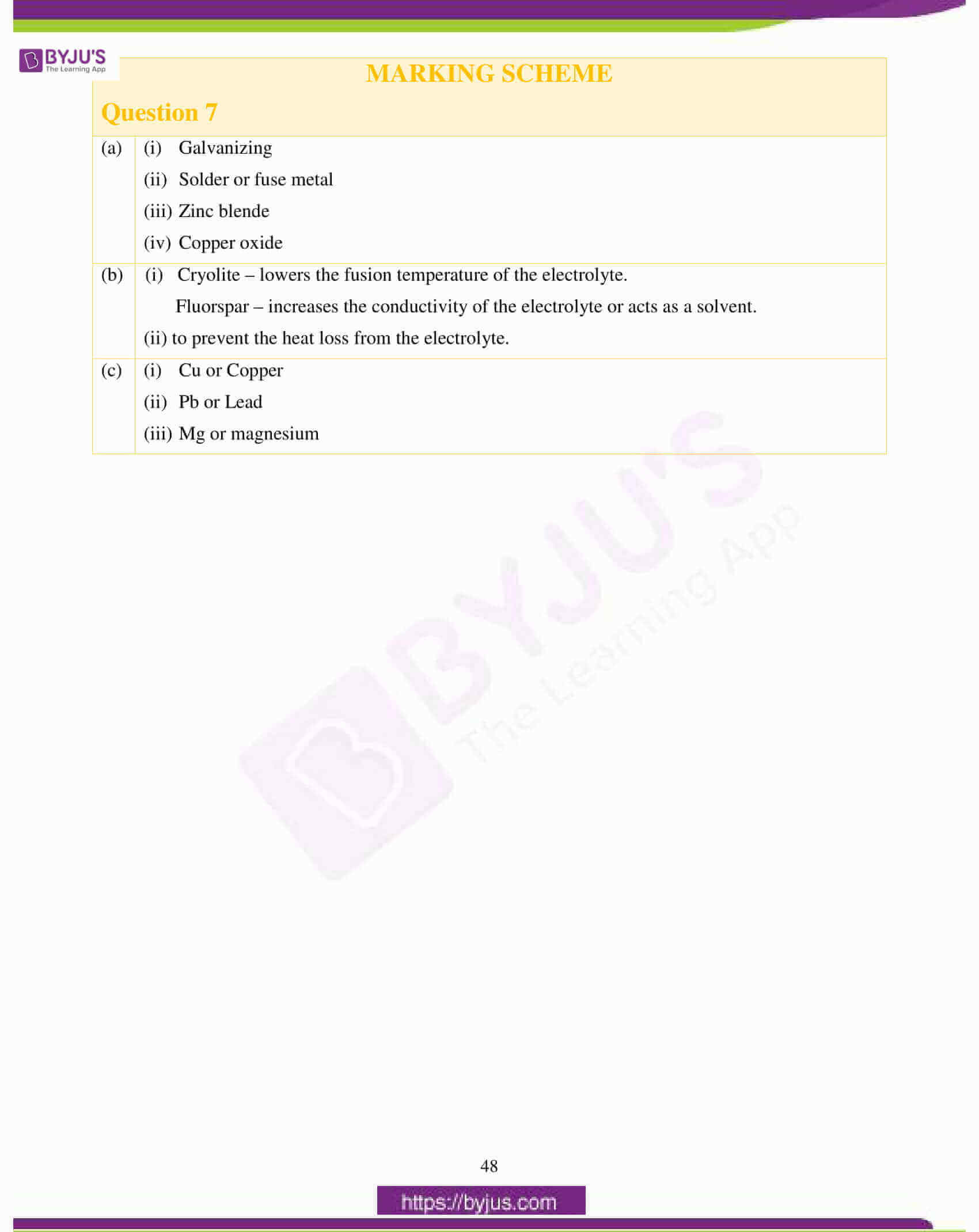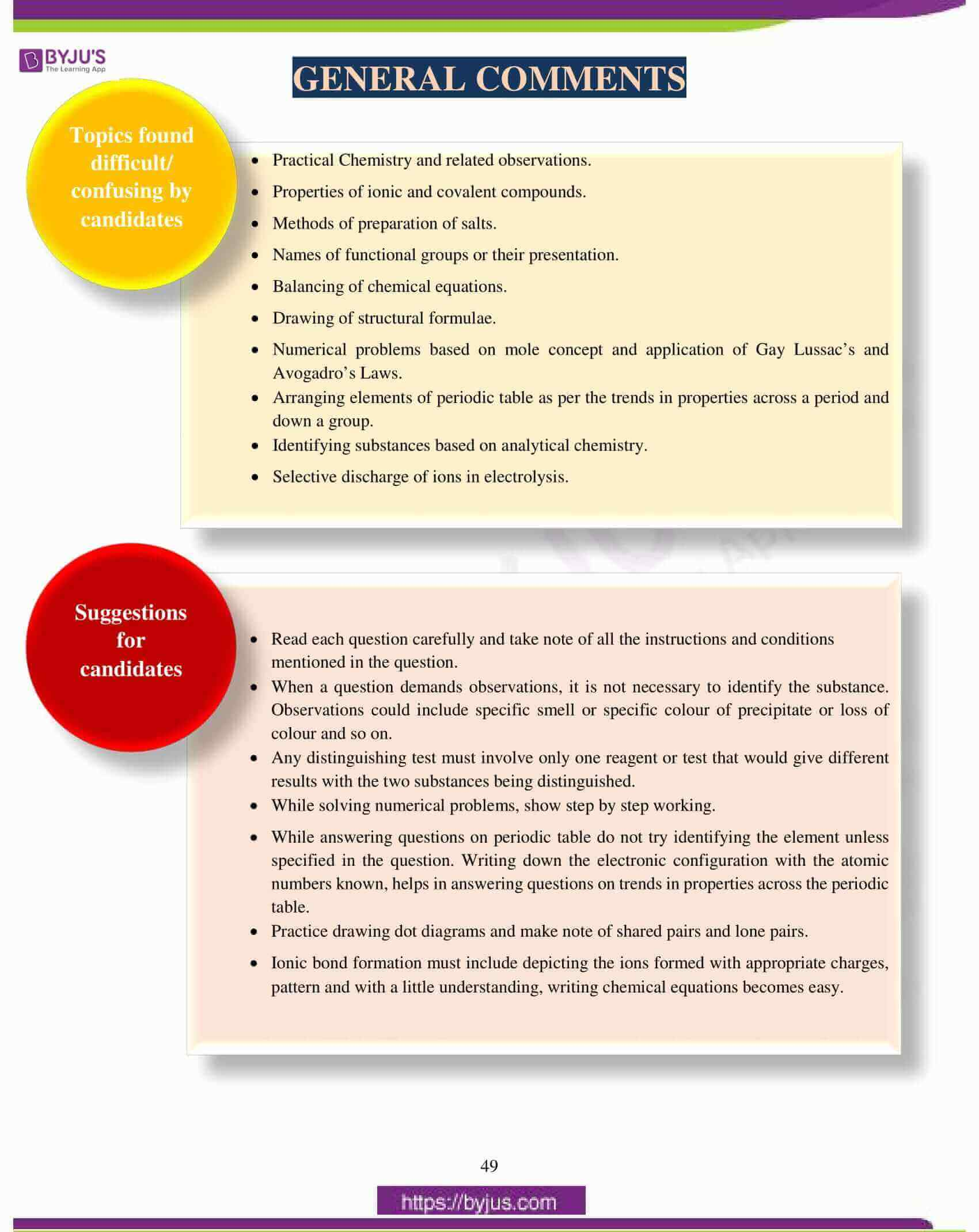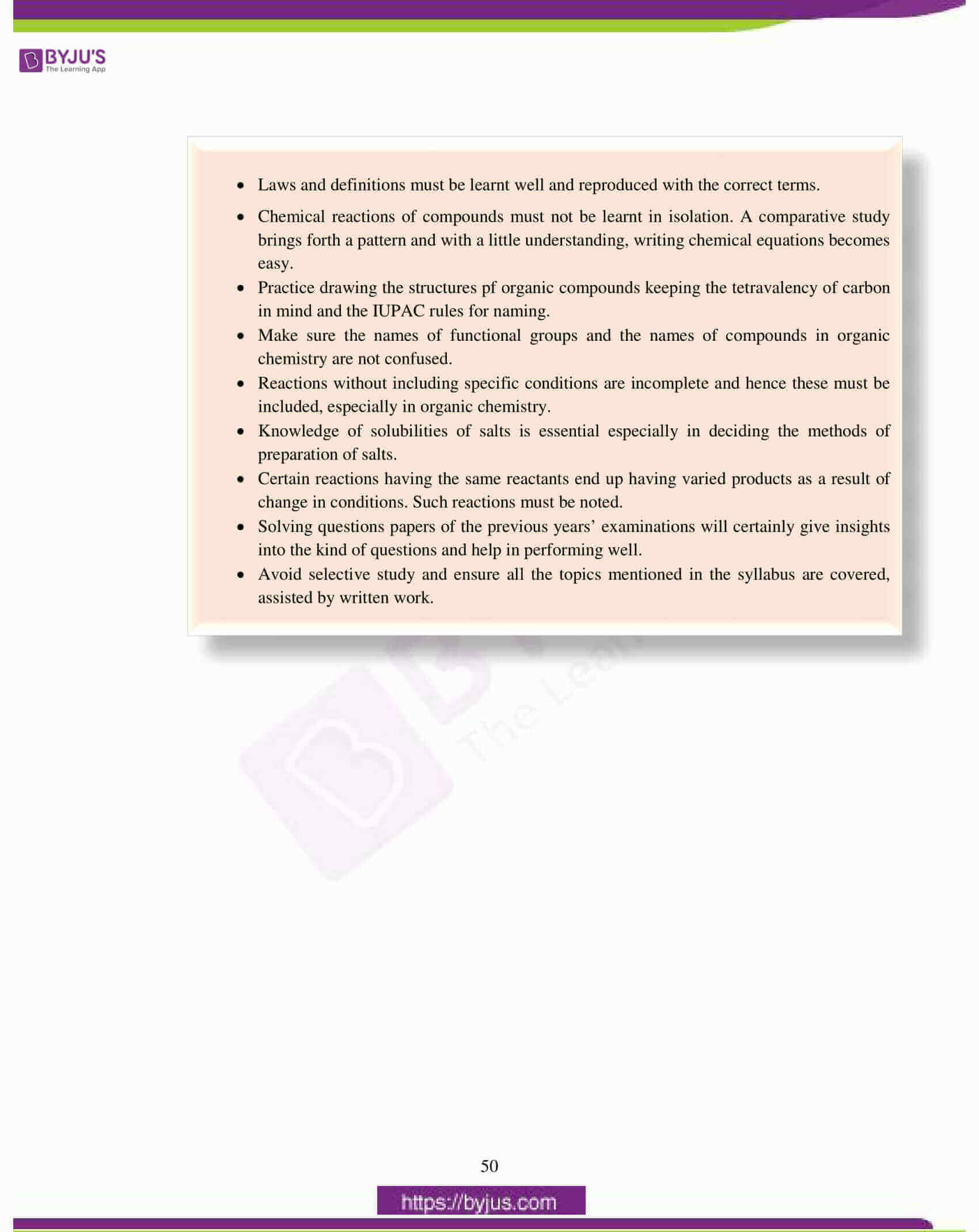## ICSE Class 10 Chemistry (Science Paper 2) Question Paper 2017 With Solution

### Question 1:

(a) Fill in the blanks from the choices given in brackets:

(i) The energy required to remove an electron from a neutral isolated gaseous atom and convert it into a positively charged gaseous ion is called _______________.

(electron affinity, ionisation potential, electronegativity)

(ii) The compound that does not have a lone pair of electrons is _______________.

(water, ammonia, carbon tetra chloride)

(iii) When a metallic oxide is dissolved in water, the solution formed has a high concentration of _______________ ions. (H+, H3O+, OH)

(iv) Potassium sulphite on reacting with hydrochloric acid releases ______________ gas. (Cl2, SO2, H2S)

(v) The compound formed when ethene reacts with Hydrogen is _______________. (CH4, C2H6, C3H8)

(b) Choose the correct answer from the options given below:

(i) A chloride which forms a precipitate that is soluble in excess of ammonium hydroxide, is:

1. Calcium chloride
2. Ferrous chloride
3. Ferric chloride
4. Copper chloride

(ii) If the molecular formula of an organic compound is C10H18 it is:

1. alkene
2. alkane
3. alkyne
4. Not a hydrocarbon

(iii) Which of the following is a common characteristic of a covalent compound?

1. high melting point
2. consists of molecules
3. always soluble in water
4. conducts electricity when it is in the molten state

(iv) To increase the pH value of a neutral solution, we should add:

1. an acid
2. an acid salt
3. an alkali
4. a salt

(v) Anhydrous iron(III) chloride is prepared by:

1. direct combination
2. simple displacement
3. decomposition
4. neutralization

(c) Identify the substance underlined, in each of the following cases:

(i) Cation that does not form a precipitate with ammonium hydroxide but forms one with sodium hydroxide.

(ii) The electrolyte used for electroplating an article with silver.

(iii) The particles present in a liquid such as kerosene, that is a non-electrolyte.

(iv) An organic compound containing — COOH functional group.

(v) A solid formed by reaction of two gases, one of which is acidic and the other basic in nature.

(d) Write a balanced chemical equation for each of the following:

(i) Action of cold and dilute Nitric acid on Copper.

(ii) Reaction of Ammonia with heated copper oxide.

(iii) Preparation of methane from iodomethane.

(iv) Action of concentrated sulphuric acid on Sulphur.

(v) Laboratory preparation of ammonia from ammonium chloride.

(e) State one relevant observation for each of the following reactions:

(i) Addition of ethyl alcohol to acetic acid in the presence of concentrated Sulphuric acid.

(ii) Action of dilute Hydrochloric acid on iron (II) sulphide.

(iii) Action of Sodium hydroxide solution on ferrous sulphate solution.

(iv) Burning of ammonia in air.

(v) Action of concentrated Sulphuric acid on hydrated copper sulphate.

(f) (i) Draw the structural formula for each of the following:

1. 2, 3 – dimethyl butane
2. diethyl ether
3. propanoic acid

(ii) From the list of terms given, choose the most appropriate term to match the given

description.

(calcination, roasting, pulverisation, smelting)

1. Crushing of the ore into a fine powder.
2. Heating of the ore in the absence of air to a high temperature.

(g) (i) Calculate the number of gram atoms in 4.6 grams of sodium (Na = 23).

(ii) Calculate the percentage of water of crystallization in CuSO4.5H2O

(H = 1, O = 16, S = 32, Cu = 64)

(iii) A compound of X and Y has the empirical formula XY2. Its vapour density is equal to its empirical formula weight. Determine its molecular formula.

(h) Match the atomic number 2, 4, 8, 15, and 19 with each of the following:

(i) A solid non-metal belonging to the third period.

(ii) A metal of valency 1.

(iii) A gaseous element with valency 2.

(iv) An element belonging to Group 2.

(v) A rare gas.

(a) (i) Ionisation potential

(ii) carbon tetrachloride or CCl4.

(iii) OH or hydroxyl ions

(iv) SO2 or Sulphur dioxide

(v) C2H6 or ethane

(b) (i) 4 or Copper chloride or CuCl2

(ii) 3 or alkyne

(iii) 2 or consists of molecules

(iv) 3 or an alkali

(v) 1 or direct combination

(c) (i) Ca2+ or calcium ion

(ii) Sodium argentocyanide solution Na [Ag(CN)2]

(iii) Free molecules

(iv) Carboxylic acid

(v) Ammonium chloride or NH4Cl

(d)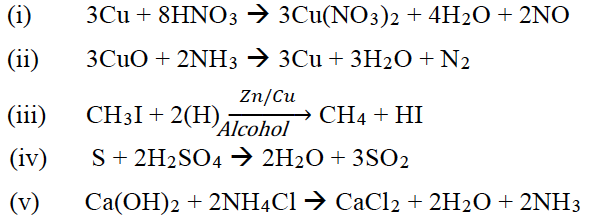(e) (i) Fruity smell evolved.

(ii) Gas with a rotten egg smell evolved.

(iii) Dirty green precipitate formed, insoluble in excess NaOH.

(iv) Burns with a green flame.

(v) Blue crystals turn into white amorphous powder.

(f)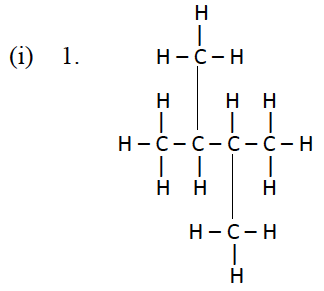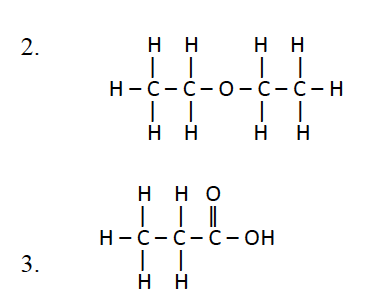(ii)

1. Pulverisation
2. Calcination

(g)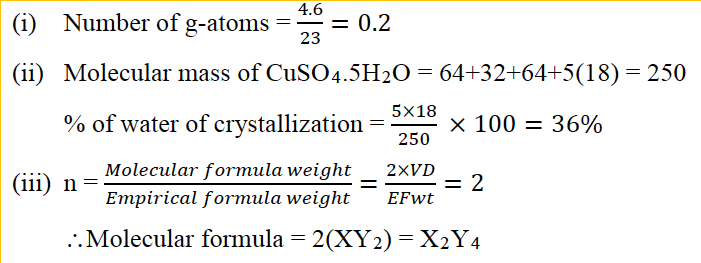(h) (i) Z = 15

(ii) Z = 19

(iii) Z = 8

(iv) Z = 4

(v) Z = 2

### Question 2:

(a) Arrange the following as per the instruction given in the brackets:

(i) He, Ar, Ne (Increasing order of the number of electron shells)

(ii) Na, Li, K (Increasing Ionisation Energy)

(iii) F, Cl, Br (Increasing electronegativity)

(iv) Na, K, Li (Increasing atomic size)

(b) State the type of Bonding in the following molecules:

(i) Water

(ii) Calcium oxide

(c) Answer the following questions:

(i) How will you distinguish between Ammonium hydroxide and Sodium hydroxide using copper sulphate solution?

(ii) How will you distinguish between dilute hydrochloric acid and dilute sulphuric acid using lead nitrate solution?

(d) Identify the salts P and Q from the observations given below:

(i) On performing the flame test salt P produces a lilac coloured flame and its solution gives a white precipitate with silver nitrate solution, which is soluble in Ammonium hydroxide solution.

(ii) When dilute HCl is added to a salt Q, a brisk effervescence is produced and the

gas turns lime water milky.

When NH4OH solution is added to the above mixture (after adding dilute HCl), it produces a white precipitate which is soluble in excess NH4OH solution

(a) (i) He, Ne, Ar

(ii) K, Na, Li

(iii) Br, Cl, F

(iv) Li, Na, K

(b) (i) Covalent bond

(ii) Ionic or electrovalent bond

(c) (i) On adding copper sulphate solution to both, the one that forms a blue precipitate that is insoluble in excess of the reagent is NaOH. The other solution forms a pale blue precipitate that dissolves in excess reagent to form an ink blue solution and this is NH4OH.

(ii) On adding lead nitrate solution to both, they form white precipitate but the one in which the white precipitate disappears on heating is HCl, while in H2SO4, the white precipitate remains insoluble on heating.

(d) (i) KCl or potassium chloride

(ii) ZnCO3 or Zinc carbonate

### Question 3:

(a) Draw an electron dot diagram to show the formation of each of the following compounds:

(i) Methane

(ii) Magnesium Chloride

[H = 1, C = 6, Mg = 12, Cl = 17]

(b) State the observations at the anode and at the cathode during the electrolysis of:

(i) fused lead bromide using graphite electrodes.

(ii) copper sulphate solution using copper electrodes.

(c) Select the ion in each case, that would get selectively discharged from the aqueous mixture of the ions listed below:

(i) SO42–, NO3 and OH

(ii) Pb2+, Ag+ and Cu2+

(a)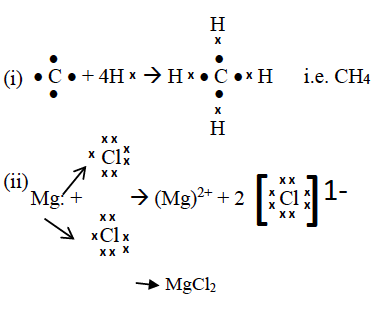(b) (i) Reddish brown fumes at the anode

Silvery grey deposit at the cathode

(ii) Size of the anode decreases or anode gets consumed

Reddish brown deposit of copper at the cathode.

(c) (i) OH ions

(ii) Ag+ ions

### Question 4:

(a) Certain blank spaces are left in the following table and these are labelled as A, B, C,

D and E. Identify each of them.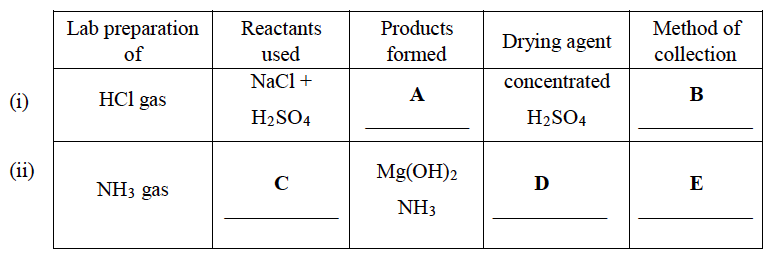(b) Write balanced chemical equations to show:

(i) The oxidizing action of concentrated Sulphuric acid on Carbon.

(ii) The behavior of H2SO4 as an acid when it reacts with Magnesium.

(iii) The dehydrating property of concentrated Sulphuric acid with sugar.

(c) Write balanced chemical equations to show how SO3 is converted to Sulphuric acid in the contact process.

(a) A – NaHSO4 + HCl

B – upward displacement of air C – Mg3N2 + H2O

D – Quicklime or CaO

E – downward displacement of air

(b)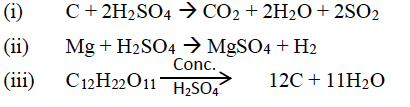(c)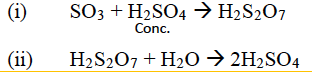### Question 5:

(a) (i) Propane burns in air according to the following equation:

C3H8 + 5O2 → 3CO2 + 4H2O.

What volume of propane is consumed on using 1000 cm3 of air, considering only

20% of air contains oxygen?

(ii) The mass of 11.2 litres of a certain gas at s.t.p. is 24 g. Find the gram molecular mass of the gas.

(b) A gas cylinder can hold 1 kg of hydrogen at room temperature and pressure:

(i) Find the number of moles of hydrogen present.

(ii) What weight of CO2 can the cylinder hold under similar conditions of temperature and pressure? (H= 1, C = 12, O = 16)

(iii) If the number of molecules of hydrogen in the cylinder is X, calculate the number of CO2

molecules in the cylinder under the same conditions of temperature and pressure.

(iv) State the law that helped you to arrive at the above result.

(c) Write a balanced chemical equation for the preparation of each of the following salts:

(i) Copper carbonate

(ii) Ammonium sulphate crystals

(a)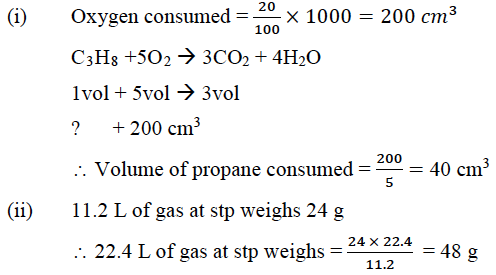(b) (i) Number of moles of hydrogen = 1000/2 = 500

(ii) Mass of CO2 = 500 × (12 + 32) = 500 × 44 = 22000 g = 22 kg

(iii) Number of molecules of CO2 = X

(iv) According to Avogadro’s law, equal volumes of all gases contain the same number of molecules under the same conditions of temperature and pressure.

(c) (i) Any soluble salt of copper reacted with sodium, potassium or ammonium carbonate solution

CuCl2 + Na2CO3 → CuCO3 + 2NaCl

(ii) 2NH4OH + H2SO4 → (NH4)2SO4 + 2H2O

### Question 6:

(a) Give a balanced chemical equation for each of the following:

(i) Action of concentrated Nitric acid on Sulphur.

(ii) Catalytic oxidation of Ammonia.

(iii) Laboratory preparation of Nitric acid.

(iv) Reaction of Ammonia with Nitric acid.

(b) Identify the term or substance based on the descriptions given below:

(i) Ice like crystals formed on cooling an organic acid sufficiently.

(ii) Hydrocarbon containing a triple bond used for welding purposes.

(iii) The property by virtue of which the compound has the same molecular formula but different structural formulae.

(iv) The compound formed where two alkyl groups are linked by group.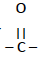(c) Give a balanced chemical equation for each of the following:

(i) Preparation of ethane from Sodium propionate

(ii) Action of alcoholic KOH on bromoethane.

(a)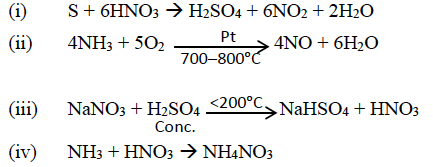(b) (i) glacial acetic acid

(ii) acetylene or ethyne

(iii) Isomerism

(iv) Ketone

(c)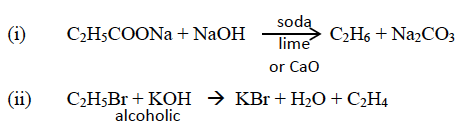### Question 7:

(a) Name the following:

(i) The process of coating of iron with zinc.

(ii) An alloy of lead and tin that is used in electrical circuits.

(iii) An ore of zinc containing its sulphide.

(iv) A metal oxide that can be reduced by hydrogen.

(b) Answer the following questions with respect to the electrolytic process in the extraction of aluminum:

(i) Identify the components of the electrolyte other than pure alumina and the role

played by each.

(ii) Explain why powdered coke is sprinkled over the electrolytic mixture.

(c) Complete the following by selecting the correct option from the choices given:

(i) The metal which does not react with water or dilute H2SO4 but reacts with

concentrated H2SO4 is _______________. (Al/Cu/Zn/Fe)

(ii) The metal whose oxide, which is amphoteric, is reduced to metal by carbon reduction _______________. (Fe/Mg/Pb/Al)

(iii) The divalent metal whose oxide is reduced to metal by electrolysis of its fused salt is _______________. (Al/Na/Mg/K).

(a) (i) Galvanizing

(ii) Solder or fuse metal

(iii) Zinc blende

(iv) Copper oxide

(b) (i) Cryolite – lowers the fusion temperature of the electrolyte.

Fluorspar – increases the conductivity of the electrolyte or acts as a solvent.

(ii) to prevent the heat loss from the electrolyte.

(c) (i) Cu or Copper

(ii) Pb or Lead

(iii) Mg or magnesium

We hope ICSE Class 10 Chemistry Question Paper Solution 2017 must have helped students in their exam preparation. We have also compiled the solutions of other subjects of ICSE Class 10 Previous Years Question Papers at one place. Students can access them by clicking here. Stay tuned to BYJU’S for the latest update on ICSE/CBSE/State Boards/Competitive exams and don’t forget to download the BYJU’S App.